# 14 TEMPORAL GRAPH NETWORKS FOR DEEP LEARNING ON DYNAMIC GRAPHS

## Figure and table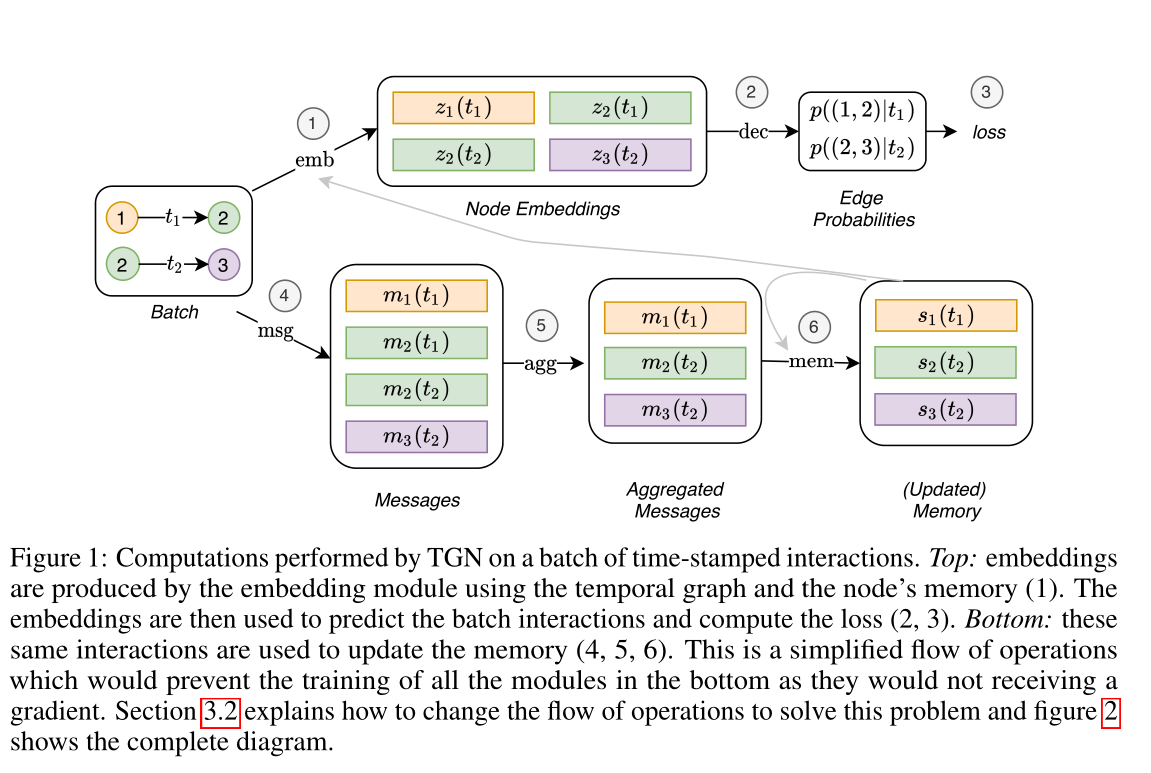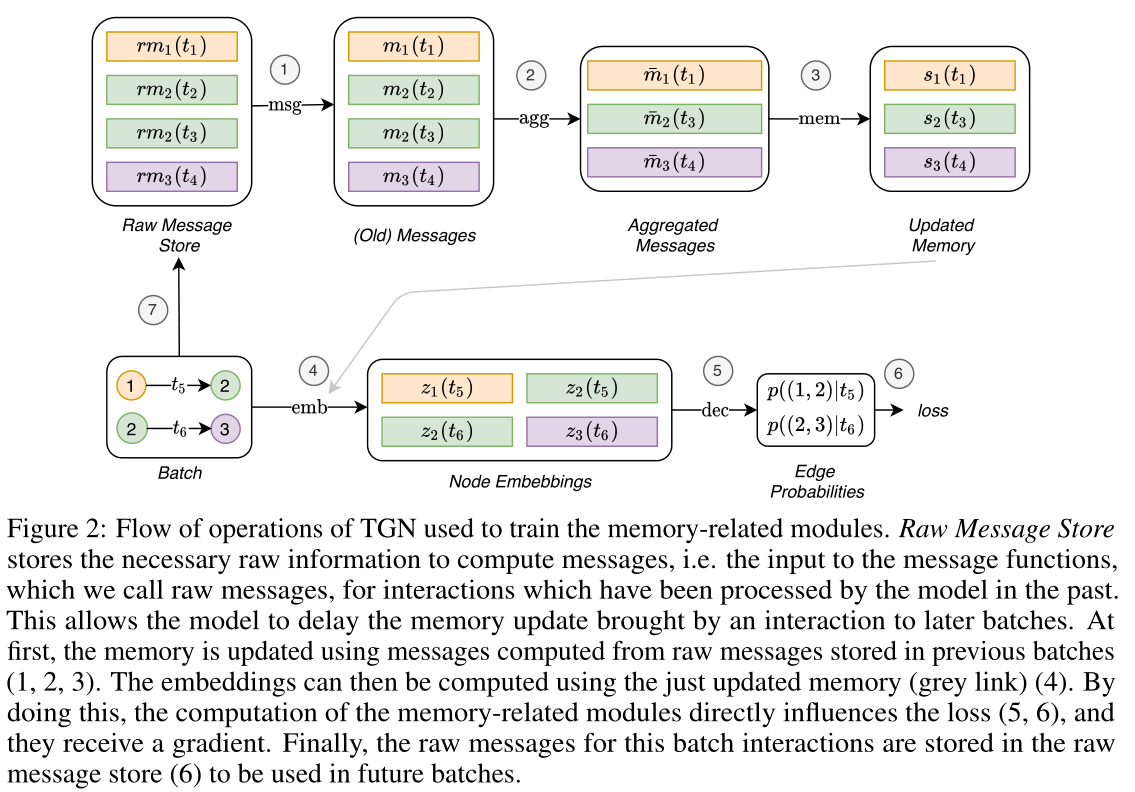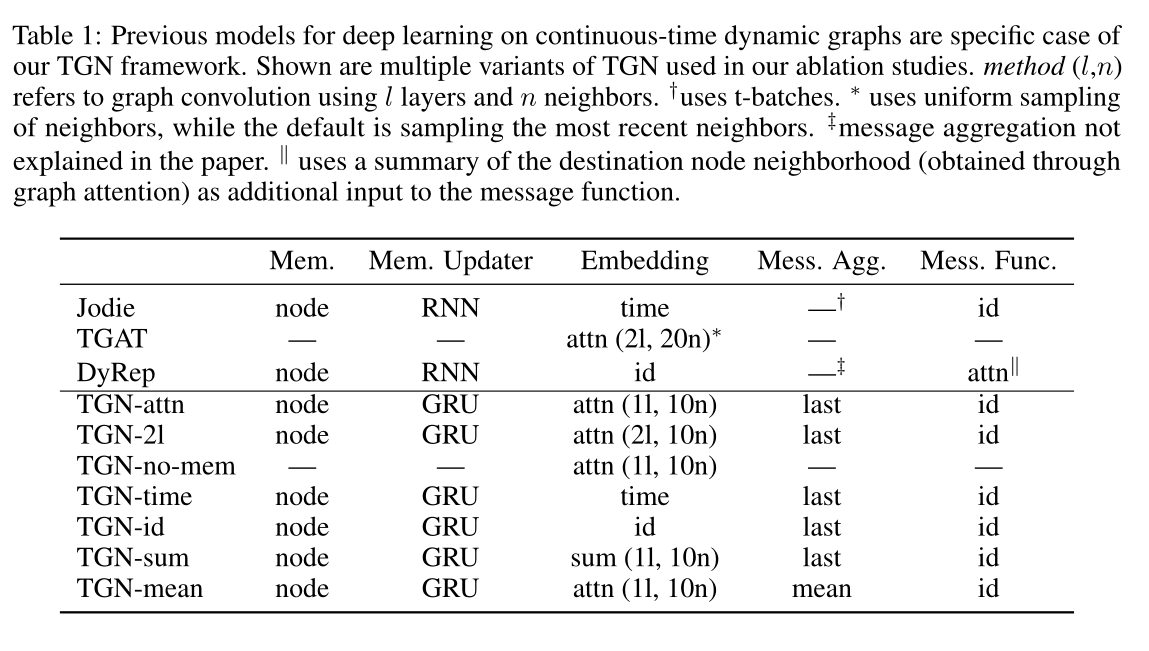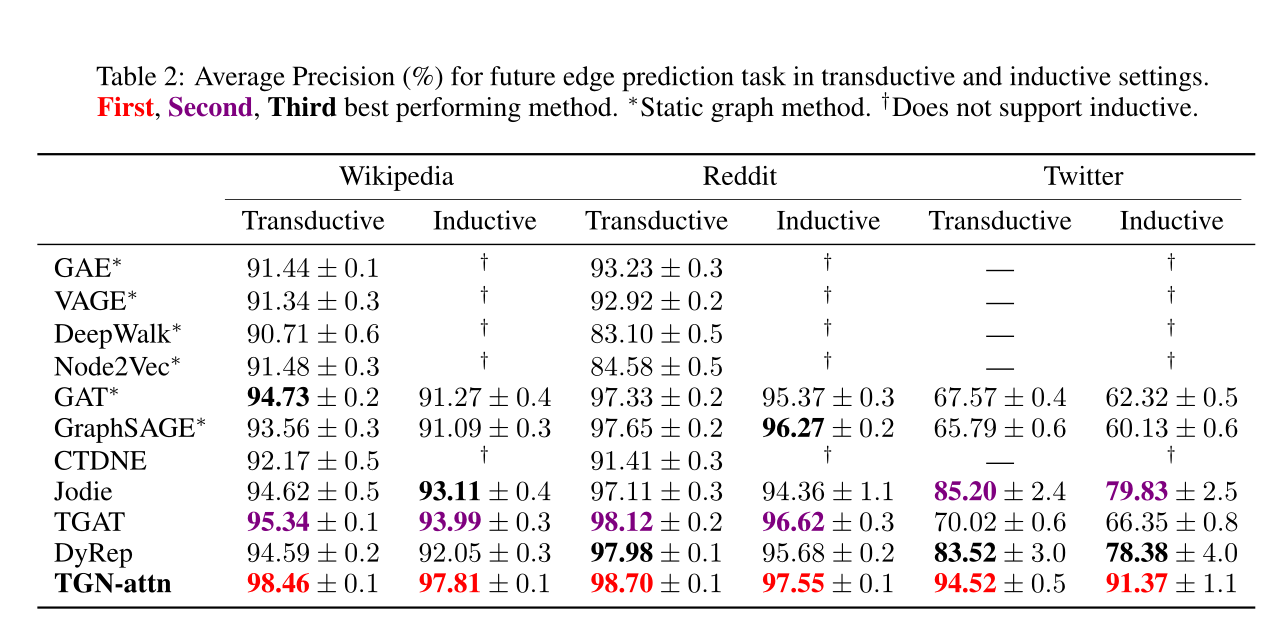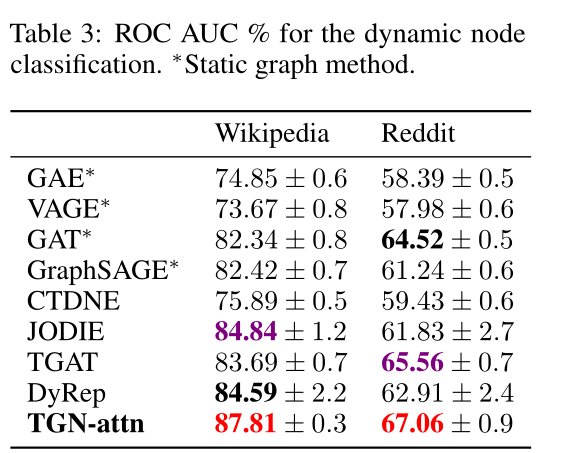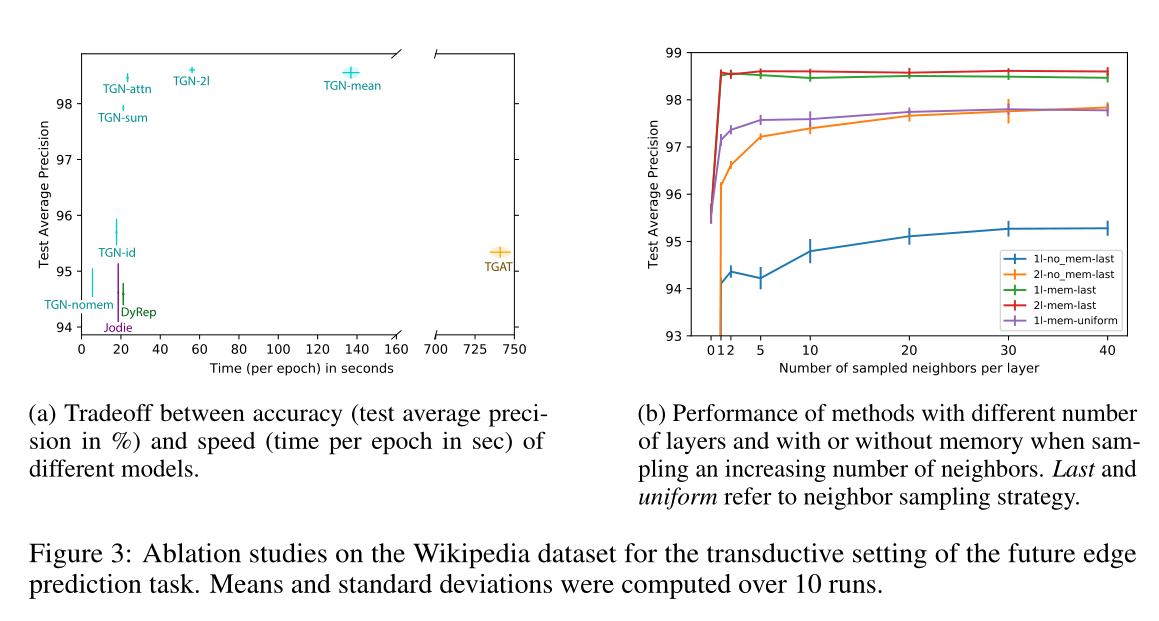(a)不同模型的精度(以%为单位的测试平均精度)和速度(以秒为单位的epoch时间)之间的权衡。

(b)随着邻居数量的增加，不同层数、有无记忆的方法的性能。最后统一采用邻居抽样策略。## Method

### 2 BACKGROUND

$\mathbf{z}_{i}=\sum_{j \in n_{i}} h\left(\mathbf{m}_{i j}, \mathbf{v}_{i}\right) \quad \mathbf{m}_{i j}=\operatorname{msg}\left(\mathbf{v}_{i}, \mathbf{v}_{j}, \mathbf{e}_{i j}\right)$

1)节点事件由$$\mathbf{v}_i(t)$$表示，其中$$i$$表示节点的索引，$$\mathbf{v}$$是与事件相关的向量属性。如果索引$$i$$以前没有出现过，事件创建节点$$i$$(带有给定的特征)，否则它更新特征。

2)节点$$i$$$$j$$之间的交互事件用一个(有向的)时间边$$\mathbf{e}_{ij}(t)$$表示(在一对节点之间可能有不止一条边，所以技术上这是一个多重图)。

### 3 TEMPORAL GRAPH NETWORKS

3.1 CORE MODULES

$\mathbf{m}_{i}(t)=\operatorname{msg}_{\mathrm{s}}\left(\mathbf{s}_{i}\left(t^{-}\right), \mathbf{s}_{j}\left(t^{-}\right), \Delta t, \mathbf{e}_{i j}(t)\right), \quad \mathbf{m}_{j}(t)=\operatorname{msg}_{\mathrm{d}}\left(\mathbf{s}_{j}\left(t^{-}\right), \mathbf{s}_{i}\left(t^{-}\right), \Delta t, \mathbf{e}_{i j}(t)\right)$

$\mathbf{m}_{i}(t)=\operatorname{msg}_{\mathrm{n}}\left(\mathbf{s}_{i}\left(t^{-}\right), t, \mathbf{v}_{i}(t)\right)$

$$\operatorname{msg}_{\mathrm{s}},\operatorname{msg}_{\mathrm{d}},\operatorname{msg}_{\mathrm{n}}$$是可学的消息函数，例如MLP。在所有的实验中，为了简单起见，我们选择消息函数作为标识(id)，它只是输入的连接。该框架还支持删除事件，在附录A.1中有介绍。

$\bar{\mathbf{m}}_i(t)=\operatorname{agg}(\mathbf{m}_{i}(t_1),…,\mathbf{m}_{i}(t_b))$

$\mathbf{s}_{i}(t)=\operatorname{mem}(\bar{\mathbf{m}}_i(t),\mathbf{s}_{i}(t^{-}))$

$\mathbf{z}_{i}(t)=\operatorname{emb}(i, t)=\sum_{j \in n_{i}^{k}([0, t])} h\left(\mathbf{s}_{i}(t), \mathbf{s}_{j}(t), \mathbf{e}_{i j}, \mathbf{v}_{i}(t), \mathbf{v}_{j}(t)\right)$

\begin{aligned} \mathbf{h}_{i}^{(l)}(t) &=\operatorname{MLP}^{(l)}\left(\mathbf{h}_{i}^{(l-1)}(t) \| \tilde{\mathbf{h}}_{i}^{(l)}(t)\right) \\ \tilde{\mathbf{h}}_{i}^{(l)}(t) &=\operatorname{MultiHeadAttention~}^{(l)}\left(\mathbf{q}^{(l)}(t), \mathbf{K}^{(l)}(t), \mathbf{V}^{(l)}(t)\right) \\ \mathbf{q}^{(l)}(t) &=\mathbf{h}_{i}^{(l-1)}(t) \| \phi(0) \\ \mathbf{K}^{(l)}(t) &=\mathbf{V}^{(l)}(t)=\mathbf{C}^{(l)}(t) \\ \mathbf{C}^{(l)}(t) &=\left[\mathbf{h}_{1}^{(l-1)}(t)\left\|\mathbf{e}_{i 1}\left(t_{1}\right)\right\| \phi\left(t-t_{1}\right), \ldots, \mathbf{h}_{N}^{(l-1)}(t)\left\|\mathbf{e}_{i N}\left(t_{N}\right)\right\| \phi\left(t-t_{N}\right)\right] \end{aligned}

$$\phi(·)$$表示一种通用时间编码

$$||$$是连接操作符

$$\mathbf{z}_{i}(t)=\operatorname{emb}(i, t)=\mathbf{h}_i^{(L)}(t)$$

\begin{aligned} \mathbf{h}_{i}^{(l)}(t) &=\mathbf{W}_{2}^{(l)}\left(\mathbf{h}_{i}^{(l-1)}(t) \| \tilde{\mathbf{h}}_{i}^{(l)}(t)\right) \\ \tilde{\mathbf{h}}_{i}^{(l)}(t) &=\operatorname{ReLu}\left(\sum_{j \in n_{i}([0, t])} \mathbf{W}_{1}^{(l)}\left(\mathbf{h}_{j}^{(l-1)}(t)\left\|\mathbf{e}_{i j}\right\| \phi\left(t-t_{j}\right)\right)\right) \end{aligned}

$$\mathbf{z}_{i}(t)=\operatorname{emb}(i, t)=\mathbf{h}_i^{(L)}(t)$$

#### 3.2 TRAINING

TGN可以训练用于各种任务，如边缘预测(自我监督)或节点分类(半监督)。以链接预测为例:提供一个时间有序的相互作用列表，目标是根据过去观察到的相互作用预测未来的相互作用。图1显示了TGN对一批训练数据进行的计算。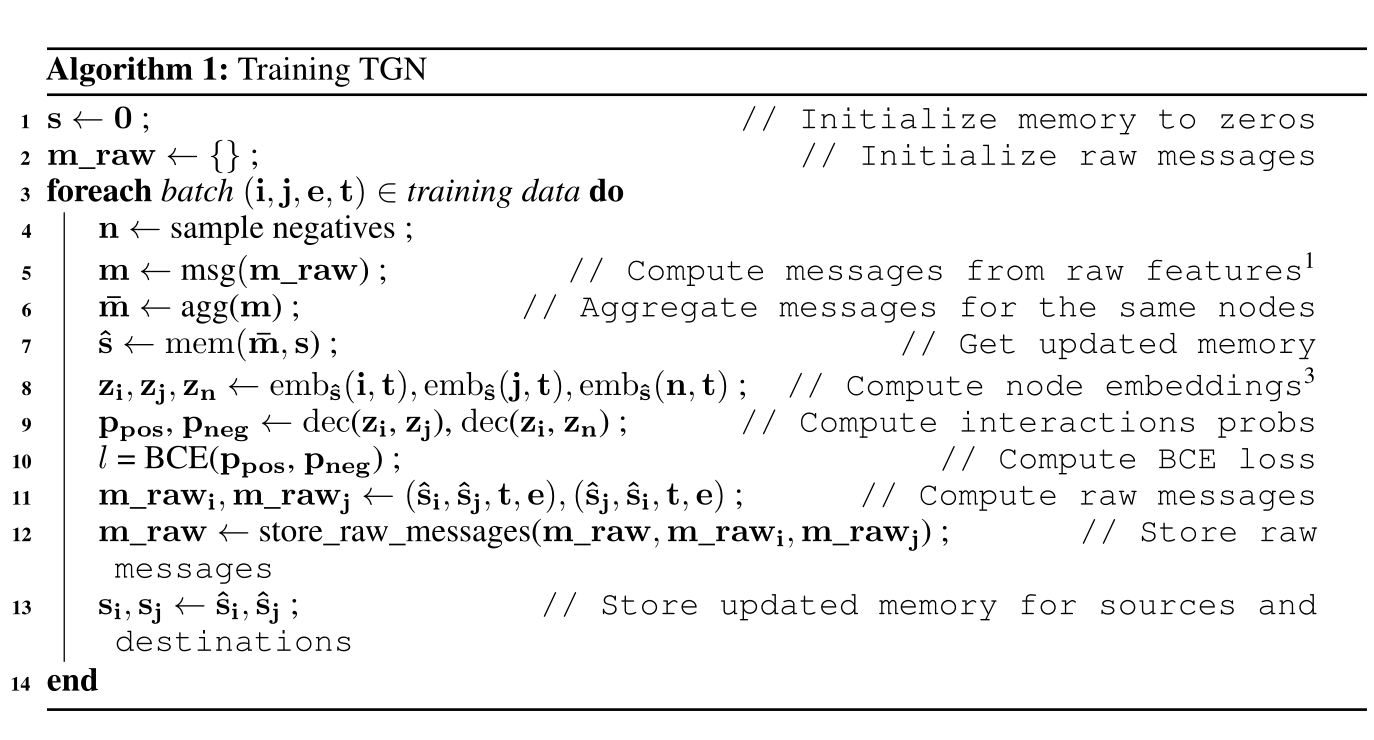## Experiment

Baselines：

CTDNE ，JODIE，DyRep ，TGAT

GAE ，VGAE ，DeepWalk，Node2Vec，GAT，GraphSAGE

sota见表2